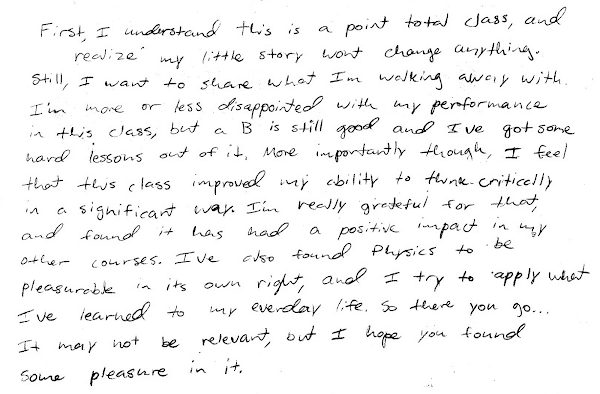## 20140111

### Physics final exam problem: metal samples placed in water

Physics 205A Final Exam, fall semester 2013
Cuesta College, San Luis Obispo, CA

Cf. Giambattista/Richardson/Richardson, Physics, 2/e, Practice Problem 14.5, Comprehensive Problem 14.95

A 0.50 kg sample of iron at 95° C and a 0.75 kg sample of aluminum at 10° C are both placed in a container with 1.00 kg of water at 20° C. Find the temperature of the water after thermal equilibrium has been reached. Ignore the effects of evaporation and phase changes, and heat exchanged with the environment and container. Show your work and explain your reasoning using the properties of heat, temperature, and thermal equilibrium.

Specific heat of iron is 440 ￼J/(kg·K). Specific heat of aluminum is 900 ￼J/(kg·K)￼. Specific heat of water is 4,190 ￼￼J/(kg·K).

• p:
Correct. Sets up an energy transfer/balance equation with changes in the thermal internal energies of the iron, aluminum and water summing to zero (no heat exchanged with container and environment), and solves for the final thermal equilibrium temperature of the system.
• r:
Nearly correct, but includes minor math errors.
• t:
Nearly correct, but approach has conceptual errors, and/or major/compounded math errors. At least has energy transfer/balance equation with three terms, but typically does not set up ∆T terms correctly.
• v:
Implementation of right ideas, but in an inconsistent, incomplete, or unorganized manner. At least some attempt at setting up change in internal energy terms and/or an energy transfer/balance equation.
• x:
Implementation of ideas, but credit given for effort rather than merit. No clear attempt at applying energy transfer/balance equation.
• y:
Irrelevant discussion/effectively blank.
• z:
Blank.
Sections 70854, 70855, 73320
Exam code: finaln0M3
p: 17 students
r: 5 students
t: 10 students
v: 12 students
x: 6 students
y: 5 students
z: 6 students

A sample "p" response (from student 5678):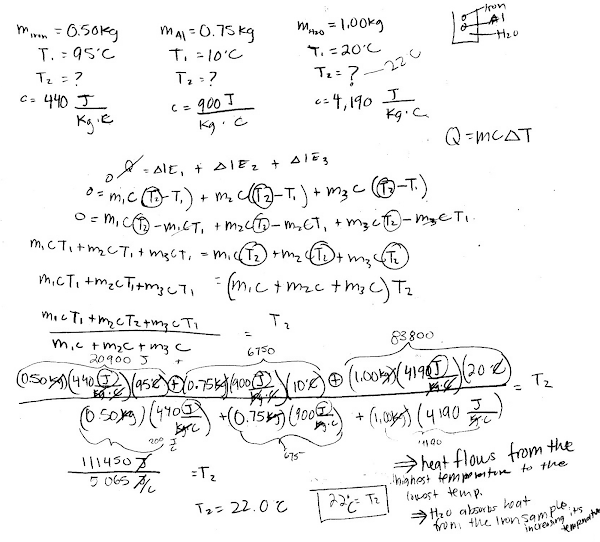A sample "p" response (from student 0618), first converting the initial temperatures to Kelvin, to find the final temperature in Kelvin: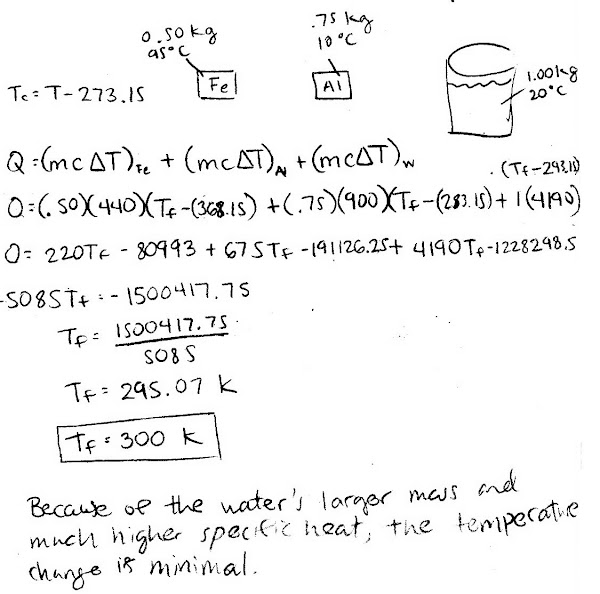A sample "t" response (from student 0817):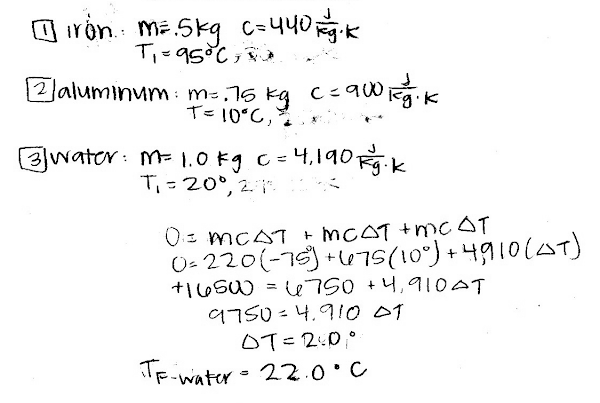A sample "v" response (from student 0716):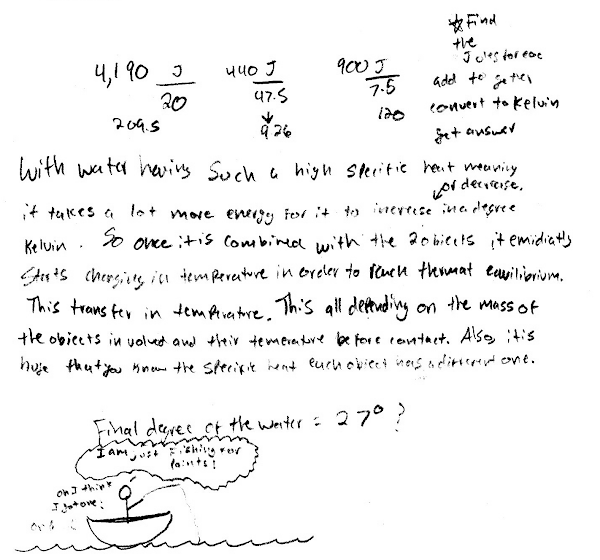A sample "y" response (from student 8207):